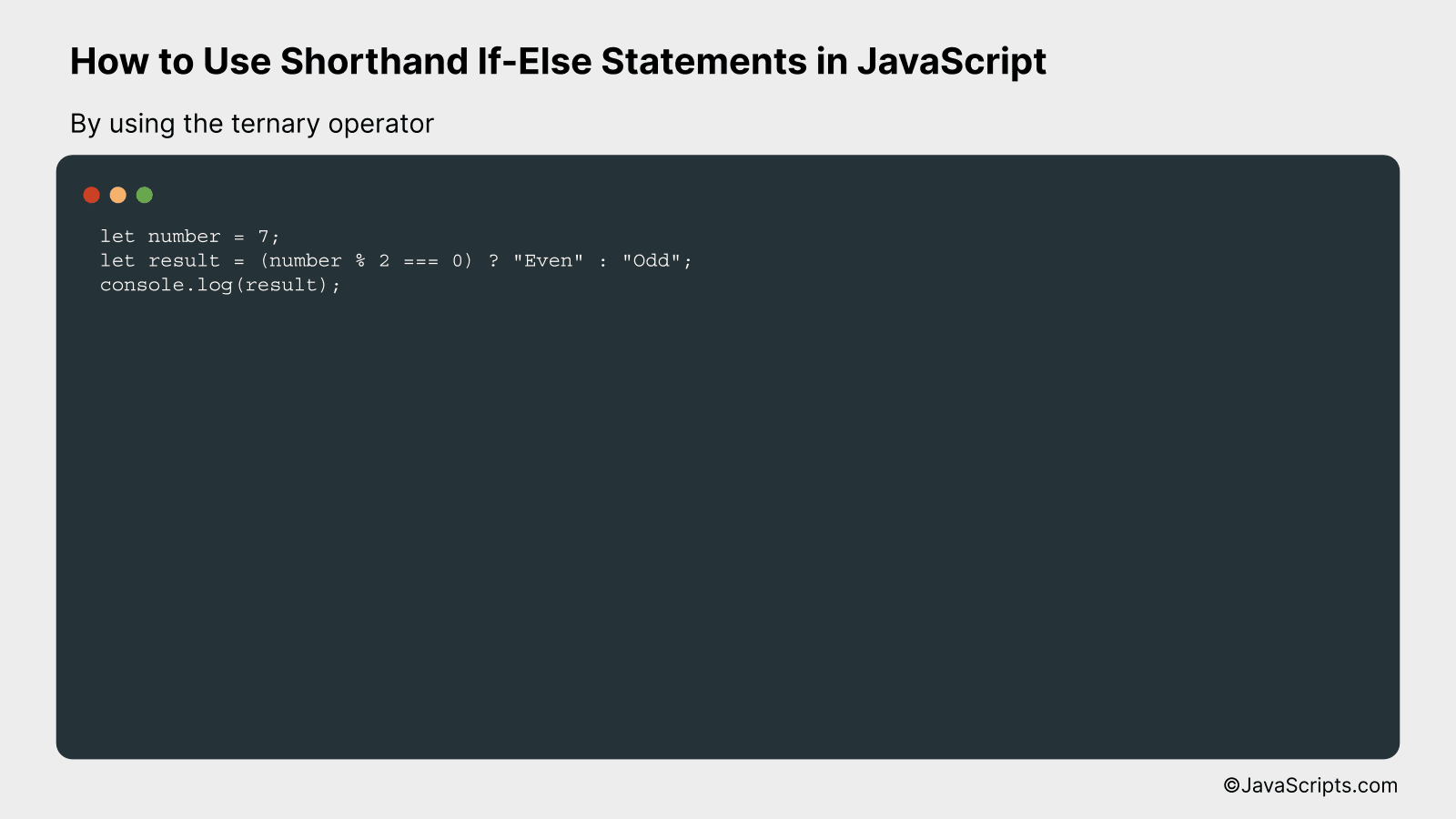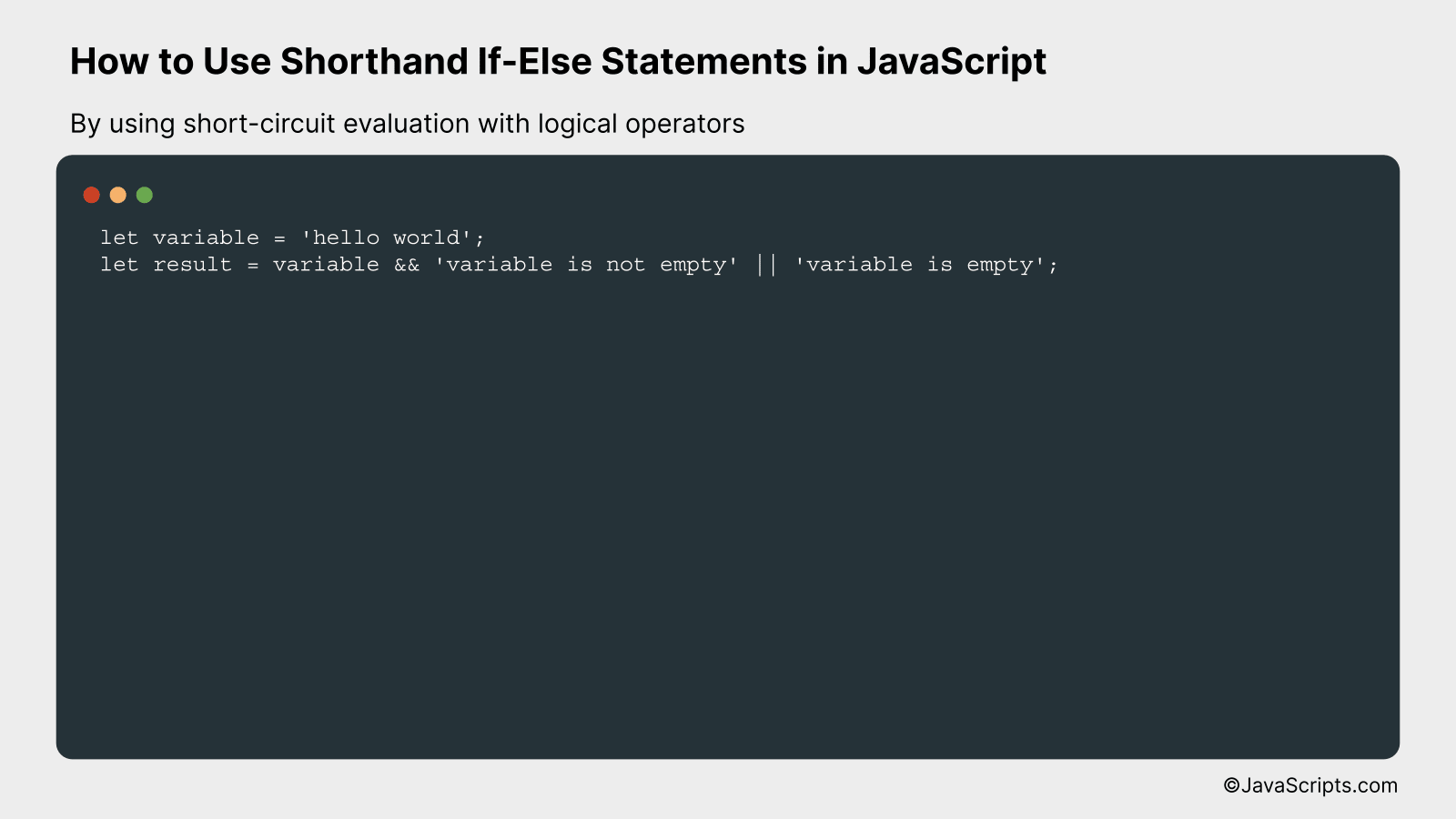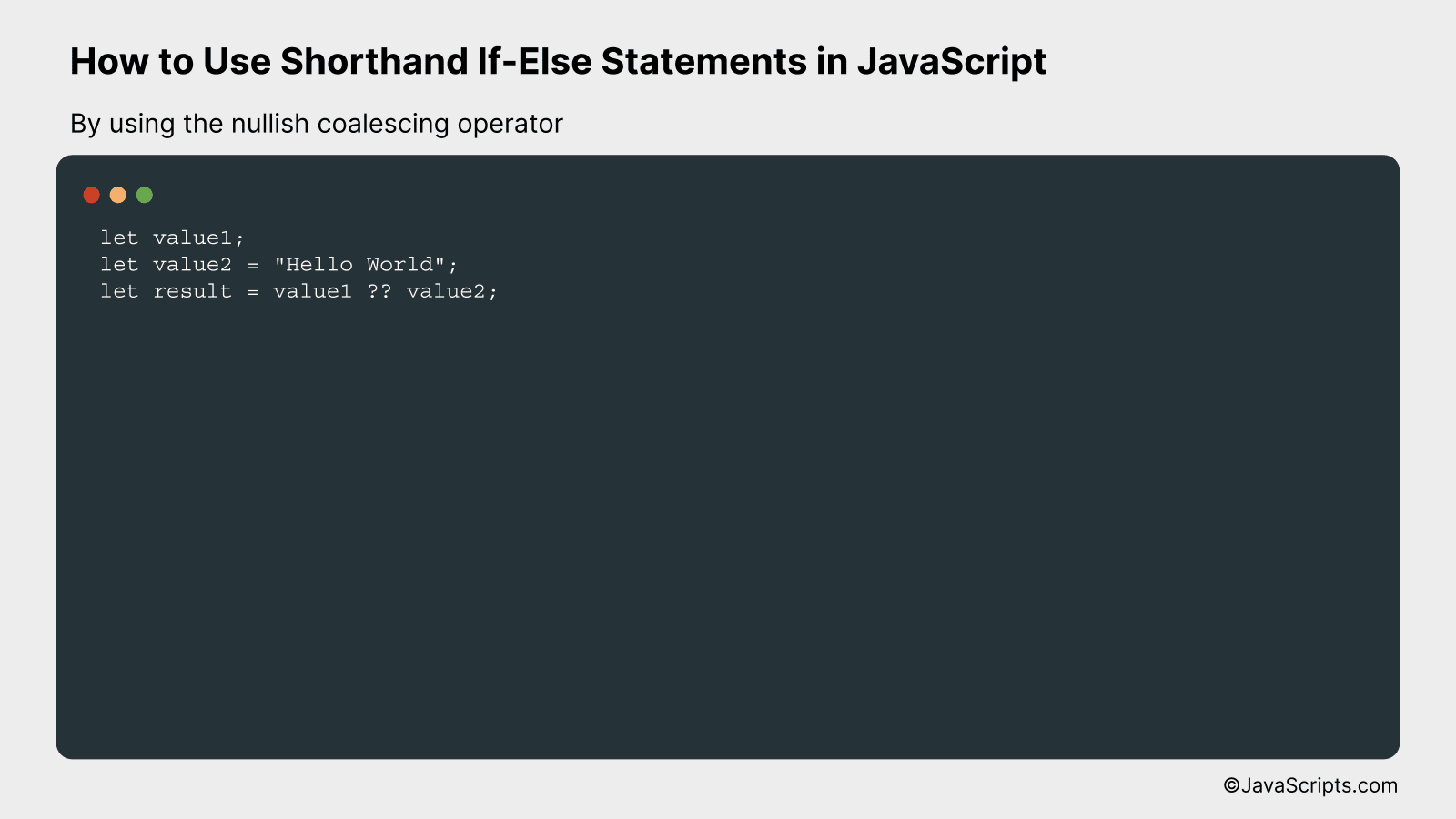# How to Use Shorthand If-Else Statements in JavaScript

JavaScript, a dynamic programming language, is the backbone of modern web development. It has greatly eased the process of creating interactive web pages, and one feature that makes it particularly powerful is the shorthand if-else statement.

Now, you might be wondering, what is a shorthand if-else statement? It’s a concise way to write if-else statements, reducing the number of lines in your code and making it easier to read and write. I’ll walk you through the nitty-gritty of this topic, ensuring you grasp the concept effortlessly.

In this post, we will be looking at the following 3 ways to use shorthand if-else statements in JavaScript:

• By using the ternary operator
• By using short-circuit evaluation with logical operators
• By using the nullish coalescing operator

Let’s explore each…

## #1 – By using the ternary operatorThe ternary operator in JavaScript is a concise way to execute if-else conditions in a single line. It follows the syntax: (condition) ? (expression if condition is true) : (expression if condition is false).

For instance, if we want to determine if a number is even or odd, we can use the ternary operator as follows:

``````
let number = 7;
let result = (number % 2 === 0) ? "Even" : "Odd";
console.log(result);
``````

### How it works

The ternary operator first evaluates the condition number % 2 === 0. If the condition is true, it will return the expression right after the ?, which is “Even” in this case. If the condition is false, it will return the expression after the : which is “Odd”.

Here’s a step-by-step breakdown of how it works:

• JavaScript first checks the condition number % 2 === 0.
• If the condition is true (i.e., the number is even), the expression after the ? is executed, and “Even” is assigned to the variable result.
• If the condition is false (i.e., the number is odd), the expression after the : is executed, and “Odd” is assigned to the variable result.
• Finally, the console.log(result) command prints the value of result to the console.

## #2 – By using short-circuit evaluation with logical operatorsJavaScript allows short-circuit evaluation with logical operators like ‘&&’ and ‘||’, which allows you to write shorthand if-else statements in a single line. When using ‘&&’, if the first operand is falsy the second operand is not evaluated, and when using ‘||’, if the first operand is truthy the second is not evaluated. This can be used to implement simple if-else conditions.

``````
let variable = 'hello world';
let result = variable && 'variable is not empty' || 'variable is empty';
``````

### How it works

In this shorthand if-else statement, JavaScript first checks the logical AND condition (variable && ‘variable is not empty’). If ‘variable’ is truthy (in JavaScript terms, it exists, is not null, not undefined, not an empty string, not zero, and not NaN), then the second operand of AND is returned. If it’s falsy, then JavaScript does not evaluate the second operand of the AND condition and moves to the OR condition, returning the string ‘variable is empty’.

• At first, JavaScript checks “variable && ‘variable is not empty'”.
• If ‘variable’ is truthy, then ‘variable is not empty’ is returned.
• If ‘variable’ is falsy, JavaScript does not check ‘variable is not empty’ and skips to the next part of the condition.
• The next part is ‘|| ‘variable is empty”. Since ‘variable’ was falsy, this string is returned.
• If ‘variable’ was truthy, JavaScript would not check this part of the condition, so this string would not be returned.

## #3 – By using the nullish coalescing operatorThe nullish coalescing operator (??) in JavaScript returns the first argument if it’s not null or undefined; otherwise, it returns the second argument, thus providing a quick shorthand for if-else statements.

Here’s an example:

``````
let value1;
let value2 = "Hello World";
let result = value1 ?? value2;
``````

### How it works

The nullish coalescing operator checks if the first value is null or undefined. If it is, it returns the second value; if not, it returns the first value. In the example above, since ‘value1’ is undefined, ‘result’ will be assigned the value of ‘value2’, i.e. “Hello World”.

• First, the variables ‘value1’ and ‘value2’ are declared. ‘value1’ is undefined and ‘value2’ is assigned the string “Hello World”.
• Then, the nullish coalescing operator (??) is used to assign a value to the ‘result’ variable.
• The operator first checks the value of ‘value1’. Since it is undefined, it moves on to ‘value2’.
• Since ‘value2’ is defined and not null, its value (“Hello World”) is returned and assigned to ‘result’.
• If ‘value1’ had a value other than null or undefined, that would be assigned to ‘result’ instead.

Related:

To wrap things up, shorthand If-Else statements are a neat way to streamline your JavaScript code, making it more compact and easier to read. Effectively using these can lead to cleaner, more efficient coding practices.

However, remember that clarity should not be sacrificed for brevity. Ensure your code is understandable, not just succinct. Shorthand If-Else statements are a tool, use them wisely.